# GSEB Solutions Class 11 Maths Chapter 6 Linear Inequalities Miscellaneous Exercise

Gujarat Board GSEB Textbook Solutions Class 11 Maths Chapter 6 Linear Inequalities Miscellaneous Exercise Textbook Questions and Answers.

## Gujarat Board Textbook Solutions Class 11 Maths Chapter 6 Linear Inequalities Miscellaneous Exercise

Solve the inequalities in questions 1 to 6:
1. 2 ≤ 3x – 4 ≤ 5
2. 6 ≤ – 3(2x – 4) ≤ 12
3. – 3 ≤ 4 – $$\frac{7x}{2}$$ ≤ 18
4. – 15 ≤ $$\frac{3(x – 2)}{5}$$ ≤ 0
5. – 12 ≤ 4 – $$\frac{3x}{- 3}$$ ≤ 2
6. 7 ≤ $$\frac{3x+11}{2}$$ ≤ 11
Solutions to questions 1 – 6:
1. 2 ≤ 3x – 4 ≤ 5
2 + 4 ≤ 3x – 4 + 4 ≤ 5 + 4
or 6 ≤ 3x ≤ 9.
Dividing by 3, we get
2 ≤ x ≤ 3.
Thus, the solution is {2, 3}2. 6 ≤ – 3(2x – 4) ≤ 12
or $$\frac{6}{3}$$ ≤ – (2x – 4) ≤ $$\frac{12}{3}$$ [Divide by 3]
or 2 ≤ – (2x – 4) ≤ 4
or 2 ≤ – 2x + 4 ≤ 4
or 2 – 4 ≤ – 2x + 4 – 4 ≤ 4 – 4
or – 2 ≤ – 2x ≤ – 0
or $$\frac{- 2}{- 2}$$ > $$\frac{- 2x}{- 2}$$ ≥ 0 [Divide by – 2]
or 1 ≥ x ≥ 0.
Hence, x is less than or equal to ‘1’ and is greater than 0, i.e; x ∈ [0, 1].

3. – 3 ≤ 4 – $$\frac{7x}{2}$$ ≤ 18
Subtracting 4, we get
– 3 – 4 ≤ 4 – $$\frac{7x}{2}$$ – 4 ≤ 18 – 4
or – 7 ≤ – $$\frac{7x}{2}$$ ≤ 14.
Dividing by – $$\frac{7}{2}$$, we get
– 7 × (- $$\frac{2}{7}$$) ≥ $$\frac{- 7}{2}$$x × (- $$\frac{2}{7}$$) ≥ 14 × (- $$\frac{2}{7}$$).
2 ≥ x ≥ – 4 or – 4 ≤ x ≤ 2 or [- 4, 2]
∴ x is less than or equal to 2 and greater than or equal to – 4.4. – 15 < $$\frac{3(x – 2)}{5}$$ ≤ 0
– 15 × 5 < $$\frac{3(x – 2)}{5}$$ × 5 ≤ 0 × 5 [Multiplying by 5]
or – 75 < 3(x – 2) ≤ 0
or – $$\frac{75}{3}$$ < x – 2 ≤ $$\frac{0}{3}$$ [Divide by 3]
or – 25 < x – 2 ≤ 0
or – 25 < x – 2 ≤ 0
or – 25 + 2 < x – 2 + 2 ≤ 0 + 2 [Add 2]
or – 23 < x ≤ 2.

5. – 12 < 4 – $$\frac{3x}{- 5}$$ ≤ 2.
Subtracting 4 from each side, we get
– 12 – 4 < 4 – $$\frac{3x}{- 5}$$ – 4 ≤ 2 – 4.
or – 16 < $$\frac{3x}{5}$$ ≤ – 2.
Multiplying each side by $$\frac{5}{3}$$, we get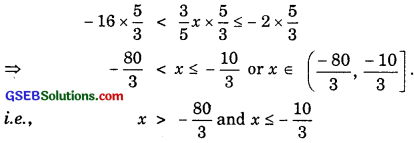6. 7 ≤ $$\frac{3x+11}{2}$$ ≤ 11.
Multiplying each side by 2, we get
7 × 2 ≤ $$\frac{3x+11}{2}$$ × 2 ≤ 11 × 2.
or 14 ≤ 3x + 11 ≤ 22.
Subtracting 11, we get
14 – 11 ≤ 3x + 11 – 11 ≤ 22 – 11.
or 3 ≤ 3x ≤ 11.
Dividing by 3, we get
1 ≤ x ≤ $$\frac{11}{3}$$ or x ∈ [1, $$\frac{11}{3}$$].
i.e; x is greater than or equal to 1 and less than or equal to $$\frac{11}{3}$$.Solve the inequalities from questions 7 to 10 and represent the solutions graphically on the number line:
7. 5x + 1 > – 24, 5x – 1 < 24
8. 2(x – 1) < x + 5, 3x + 2) > 2 – x
9. 3x – 7 > 2(x – 6), 6 – x > 11 – 2x
10. 5(2x – 7) – 3(2x + 3) ≤ 0, 2x + 19 ≤ 6x + 47
Solutions to questions from 7 to 11:
7. (i) 5x + 1 > – 24, subtracting 1, 5x > – 24 – 1
or 5x > – 25
∴ Dividing by 5, x > – 5.

(ii) 5x – 1 < 24.
∴ Adding 1, 5x < 24 + 1 = 25.
∴ Dividing by 5, x < 5. x > – 5 and x < 5 are shown graphically as follows: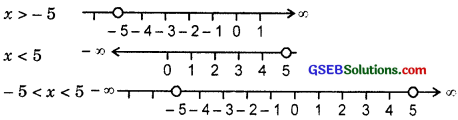i.e., x is greater than – 5 and less than 5.

8. The inequalities are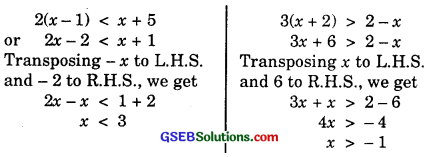Graph of the inequalities are shown below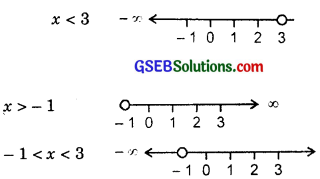i.e; x ∈ (- 1, 3) or x is greater than – 1 and less than 3.

9. The inequalities are 3x – 7 > 2(x – 6) and 6 – x > 11 – 2x.
or 3x – 7 > 2x – 12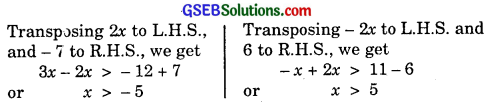Graphs of these inequalities are shown below: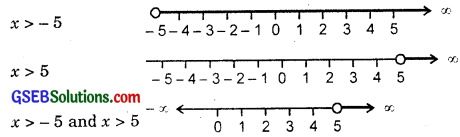x > 5 satisfies both the inequalities x > – 5 and x > 5
∴ Solution is x > 5.10. The inequalities are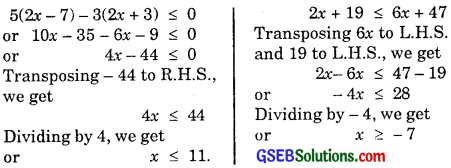Graphs of these inequalities are shown below:
x ≤ 11 i.e; x ∈ [- 7, 11]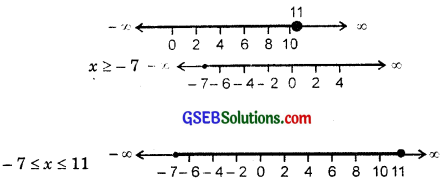Solution of x is greater than or equal to – 7 and x less or equal to 11.

11. A solution is to be kept between 68° F and 77°F. What is the range in temperature in degree Celsius (C), if the Celsius/Fahrenheit (F) conversion formula is given by
F = $$\frac{9}{5}$$C + 32
Solution:
We have:
F = $$\frac{9}{5}$$C + 32
But 68° < F < 77°.
∴ 68° < $$\frac{9}{5}$$C + 32 < 77°.
Subtracting 32 from each side
68 – 32 < $$\frac{9}{5}$$C < 77 – 32
36 < $$\frac{9}{5}$$C < 45
Multiplying by $$\frac{5}{9}$$, we get
36 × $$\frac{5}{9}$$ < C < 45 × $$\frac{5}{9}$$
20 < C < 25. 12. A solution of 8% boric acid is to be diluted by adding 2% boric acid solution to it. The resulting mixture is to be more than 4% but less than 6% boric acid. If we have 640 litres of 8% solution, how many litres of the 2% solution will have to be added? Solution: Let the 2% boric acid solution be x litres. ∴ Mixture is (640 + x) litres. or $$\frac{2x}{100}$$ + $$\frac{8 × 640}{100}$$ > $$\frac{4}{100}$$ (640 + x)
or 2x + 5120 > 2560 + 4x
or 5120 – 2560 > 4x – 2x
or 2x < 2560.
or x < 1280
Also, 2% of x + 8% of 640 < 6% of (640 + x).
or $$\frac{2x}{100}$$ + $$\frac{8×640}{100}$$ < $$\frac{6}{100}$$ (640 + x)
or 2x + 5120 < 3840 + 6x
or 5120 – 3840 < 6x – 2x or 4x > 1280 or x > 320.
Thus, the number of litres to be added should be greater than 320 litres.13. How many litres of water will have to be added to 1125 litres of the 45% solution of acid so that the resulting mixture will contain more than 25% but less than 30% acid content?
Solution:
Let x litres water is added to 45% solution of acid.
(i) ∴ 25% of (1125 + x) < $$\frac{1125×45}{100}$$
or $$\frac{1125×x}{4}$$ < $$\frac{1125×9}{20}$$.
Multiplying by 20, we get
5(1125 + x) < 10125
or 5625 + 5x < 10125.
Subtracting 5625 from both sides, we get
∴ 5x < 10125 – 5625 = 4500.
or x < $$\frac{4500}{5}$$ = 900 …………………. (1) (ii) Further 30% of (1125 + 4) > $$\frac{1125×45}{100}$$.
or $$\frac{3}{10}$$ × (1125 + x) > $$\frac{1125×45}{100}$$ > $$\frac{1125×9}{20}$$.
Multiplying both sides by 20, we get
6(1125 + x) > 1125 × 9
or 6750 + 6x > 10125.
Subtracting 6750 from both sides, we get
6x > 10125 – 6750 > 3375
or x > $$\frac{3375}{6}$$ = 562.5 ……………….. (2)
From (1) and (2), we have:
562.5 < x < 900.14. IQ of a person is given by the formula
IQ = $$\frac{MA}{CA}$$ × 100,
where MA is mental age and CA chronological age. If 80 ≤ IQ ≤ 140 for a group of 12 years old children, find the range of their mental ages.
Solution:
We have:
IQ = $$\frac{MA}{CA}$$ × 100
We are given 80 ≤ IQ ≤ 140.
Putting the values of IQ in it, we get
80 ≤ $$\frac{MA}{CA}$$ × 100 ≤ 140.
Multiplying both sides by 12, we get
12 × 80 ≤ MA × 100 ≤ 140 × 12
or 960 ≤ 100 × MA ≤ 1680.
Dividing by 100, we get
9.6 ≤ MA ≤ 16.8.
Hence, mental age is greater than or equal to 9.6 and less than or equal to 16.8 years.